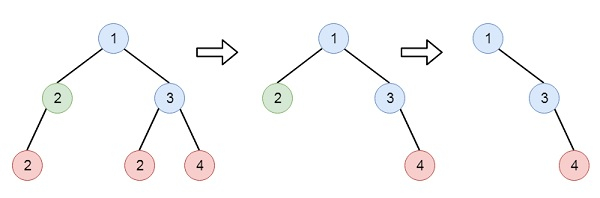# Delete Leaves With a Given Value in C++

C++Server Side ProgrammingProgramming

Suppose we have a binary tree and an integer target, we have to delete all the leaf nodes with value target. We have to keep in mind that once we delete a leaf node with a value target if it's parent node becomes a leaf node and has the value target, it should also be deleted (we need to continue doing that until we can't). So if the tree is like below, and the target is 2, then the final tree will be like the last one −To solve this, we will follow these steps −

• Define a recursive method called remLeaf(), this will take the root and the target

• if the root is null, then return null

• left := remLeaf(left of root, target)

• right := remLeaf(right of root, target)

• if the left is null and right is null and value of root is same as the target, then return null

• left of root := left

• right of root := right

• return root

## Example (C++)

Let us see the following implementation to get a better understanding −

#include <bits/stdc++.h>
using namespace std;
class TreeNode{
public:
int val;
TreeNode *left, *right;
TreeNode(int data){
val = data;
left = right = NULL;
}
};
void insert(TreeNode **root, int val){
queue<TreeNode*> q;
q.push(*root);
while(q.size()){
TreeNode *temp = q.front();
q.pop();
if(!temp->left){
if(val != NULL)
temp->left = new TreeNode(val);
else
temp->left = new TreeNode(0);
return;
} else {
q.push(temp->left);
}
if(!temp->right){
if(val != NULL)
temp->right = new TreeNode(val);
else
temp->right = new TreeNode(0);
return;
} else {
q.push(temp->right);
}
}
}
TreeNode *make_tree(vector<int> v){
TreeNode *root = new TreeNode(v);
for(int i = 1; i<v.size(); i++){
insert(&root, v[i]);
}
return root;
}
void tree_level_trav(TreeNode*root){
if (root == NULL) return;
cout << "[";
queue<TreeNode *> q;
TreeNode *curr;
q.push(root);
q.push(NULL);
while (q.size() > 1) {
curr = q.front();
q.pop();
if (curr == NULL){
q.push(NULL);
} else {
if(curr->left)
q.push(curr->left);
if(curr->right)
q.push(curr->right);
if(curr->val == 0 || curr == NULL){
cout << "null" << ", ";
} else {
cout << curr->val << ", ";
}
}
}
cout << "]"<<endl;
}
class Solution {
public:
TreeNode* removeLeafNodes(TreeNode* root, int target) {
if(!root || root->val == 0) return NULL;
TreeNode* left = removeLeafNodes(root->left, target);
TreeNode* right = removeLeafNodes(root->right, target);
if(!left && !right && root->val == target){
return NULL;
}
root->left = left;
root->right = right;
return root;
}
};
main() {
vector<int> v1 = {1,2,3,2,NULL,2,4};
TreeNode *root = make_tree(v1);
Solution ob;
tree_level_trav(ob.removeLeafNodes(root, 2));
}

## Input

[1,2,3,2,null,2,4]
2

## Output

[1, 3, 4, ]